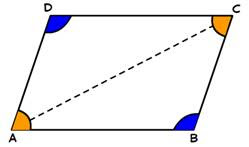## 6 April 2015

A figure formed by joining four points in order is called a quadrilateral. A quadrilateral is a closed figure having four sides, four angles and four vertices and two diagonals. The sum of four angles of a quadrilateral is 360°. Quadrilaterals can be classified into different types based on the properties of their sides and angles.

#### Trapezium

A trapezium is a quadrilateral in which one pair of opposite sides is parallel. A trapezium with equal non-parallel sides and equal base angles is known as an isosceles trapezium.

#### Kite

A kite is a quadrilateral in which two pairs of adjacent sides are equal in length and one pair of opposite angles, the ones that are between the sides of unequal length, are equal in measure and the diagonals intersect at right angles.

#### Parallelogram

A parallelogram is a quadrilateral in which, opposite sides are parallel and equal. If each pair of opposite sides of a quadrilateral are equal, then it is a parallelogram. A quadrilateral is a parallelogram if a pair of opposite sides is equal and parallel.

Opposite angles of a parallelogram are equal. If each pair of opposite angles of a quadrilateral are equal, then it is a parallelogram.

Diagonals of a parallelogram bisect each other. If the diagonals of a quadrilateral bisect each other, then it is a parallelogram. A diagonal of a parallelogram divides it into two congruent triangles. In a parallelogram, the angles are not right angles.

#### Rectangle

A rectangle is a parallelogram in which, diagonals are equal and bisect each other. It is a parallelogram in whose one angle is a right angle.

#### Square

A square is a parallelogram in which, all the sides are equal, diagonals are equal and perpendicular bisectors of each other. A square is a rectangle with equal adjacent sides.

Rhombus
A rhombus is a parallelogram in which, all the sides are equal and diagonals are perpendicular bisectors. A square is a rhombus in which, each angle measures 90°. Squares, rectangles and rhombuses are all examples of parallelograms.

### Properties of a Parallelogram

A parallelogram is a quadrilateral in which
•  opposite sides are parallel and equal,
•  opposite angles are equal,
•  the diagonals bisect each other,
•  each diagonal divides it into two congruent triangles,

A square is a parallelogram in which
•  all the sides are equal,
•  each angle measures 90°,
•  diagonals are equal and bisect at right angles.

A rectangle is a parallelogram in which
•  diagonals are equal and bisect each other,
•  each angle measures 90°.

A rhombus is a parallelogram in which
•  all the four sides are equal,
•  diagonals bisect each other at right angles.

A diagonal of a parallelogram divides it into two congruent triangles.
ABCD is a parallelogram and AC is a diagonal. ΔABC ≅ ΔCDA.

If each pair of opposite sides is equal in a quadrilateral, then it is a parallelogram.
ABCD is a quadrilateral. If AB = CD and BC = AD, then ABCD is a parallelogram.If the diagonals of a quadrilateral bisect each other, then it is a parallelogram.
ABCD is a quadrilateral and the diagonals AC and BD intersect at E.
If BE = DE and AE = CE then, ABCD is a parallelogram.

### Mid-Point Theorem

The mid-point of the line segment is the geometric centre of the line segment. Mid-point of a line segment divides it into two equal halves.

Mid-Point Theorem
The line segment joining the mid-points of two sides of a triangle is parallel to the third side and equal to half the third side.

Given: In triangle ABC, P and Q are mid-points of AB and AC respectively.

To Prove: i) PQ || BC ii) PQ = 12 BC

Construction: Draw CR || BA to meet PQ produced at R.Proof:
∠QAP = ∠QCR (Pair of alternate angles) ---------- (1)

AQ = QC ( Q is the mid-point of side AC) ---------- (2)

∠AQP = ∠CQR (Vertically opposite angles) ---------- (3)

Thus, ΔAPQ ≅ ΔCRQ (ASA Congruence rule)

PQ = QR (by CPCT) or PQ = 12 PR  ---------- (4)

⇒ AP = CR (by CPCT)  ........(5)

But, AP = BP (∵ P is the mid-point of the side AB)

⇒ BP = CR

Also. BP || CR (by construction)

In quadrilateral BCRP, BP = CR and BP || CR

Therefore, quadrilateral BCRP is a parallelogram.

BC || PR or, BC || PQ

Also, PR = BC ( BCRP is a parallelogram)

⇒ 12 PR = 12 BC

⇒ PQ = 12 BC [from (4)]

### Converse of Mid-Point Theorem

The line drawn through the mid-point of one side of a triangle and parallel to another side bisects the third side.

In triangle ABC, if P is the mid-point of AB and PQ || BC then, AQ = QC.

Quadrilateral formed by joining the mid-points of the sides of a quadrilateral, in order is a parallelogram.